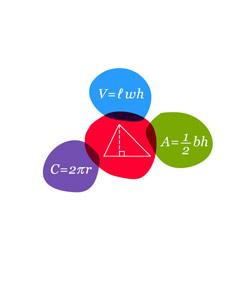# SAT Mathematics: Algebra And Simple Geometry Problems

23 QuestionsSettingsThis is a 23-question quiz that focuses strictly on algebra questions on the SAT Mathematics test. Specifically, this quiz tests your ability to solve simple linear and quadratic algebraic equations. Read the questions carefully and answer. So, let's try out the quiz. All the best!

Related Topics